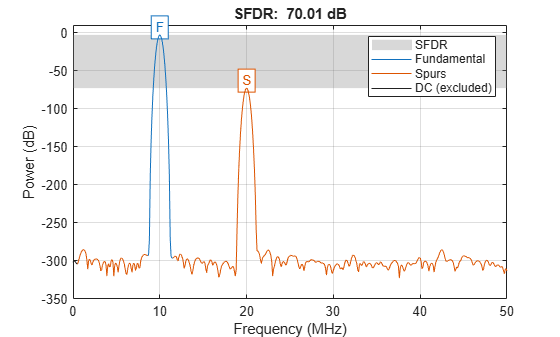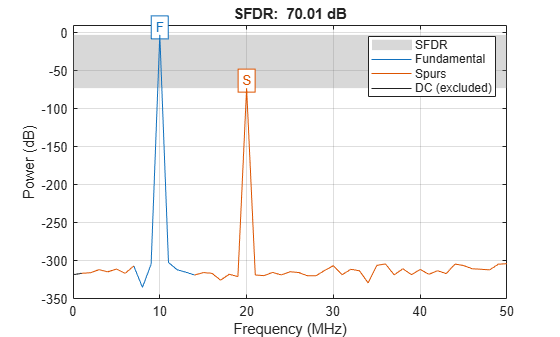# sfdr

Spurious free dynamic range

## Syntax

``r = sfdr(x)``
``r = sfdr(x,fs)``
``r = sfdr(x,fs,msd)``
``r = sfdr(sxx,f,'power')``
``r = sfdr(sxx,f,msd,'power')``
``````[r,spurpow,spurfreq] = sfdr(___)``````
``sfdr(___)``

## Description

example

````r = sfdr(x)` returns the spurious free dynamic range (SFDR), `r`, in dB of the real sinusoidal signal, `x`. `sfdr` computes the power spectrum using a modified periodogram and a Kaiser window with β = 38. The mean is subtracted from `x` before computing the power spectrum. The number of points used in the computation of the discrete Fourier transform (DFT) is the same as the length of the signal, `x`.```
````r = sfdr(x,fs)` returns the SFDR of the time-domain input signal, `x`, when the sample rate, `fs`, is specified. The default value of `fs` is 1 Hz.```

example

````r = sfdr(x,fs,msd)` returns the SFDR considering only spurs that are separated from the fundamental (carrier) frequency by the minimum spur distance, `msd`, specified in cycles/unit time. The sample rate is `fs`. If the carrier frequency is `Fc`, then all spurs in the interval (`Fc-msd`, `Fc+msd`) are ignored.```

example

````r = sfdr(sxx,f,'power')` returns the SFDR of the one-sided power spectrum of a real-valued signal, `sxx`. `f` is the vector of frequencies corresponding to the power estimates in `sxx`. The first element of `f` must equal 0. The algorithm removes all the power that decreases monotonically away from the DC bin.```
````r = sfdr(sxx,f,msd,'power')` returns the SFDR considering only spurs that are separated from the fundamental (carrier) frequency by the minimum spur distance, `msd`. If the carrier frequency is `Fc`, then all spurs in the interval (`Fc-msd`, `Fc+msd`) are ignored. When the input to `sfdr` is a power spectrum, specifying `msd` can prevent high sidelobe levels from being identified as spurs.```

example

``````[r,spurpow,spurfreq] = sfdr(___)``` returns the power and frequency of the largest spur.```

example

````sfdr(___)` with no output arguments plots the spectrum of the signal in the current figure window. It uses different colors to draw the fundamental component, the DC value, and the rest of the spectrum. It shades the SFDR and displays its value above the plot. It also labels the fundamental and the largest spur.```

## Examples

collapse all

Obtain the SFDR for a 10 MHz tone with amplitude 1 sampled at 100 MHz. There is a spur at the 1st harmonic (20 MHz) with an amplitude of $3.16×1{0}^{-4}$.

```deltat = 1e-8; fs = 1/deltat; t = 0:deltat:1e-5-deltat; x = cos(2*pi*10e6*t)+3.16e-4*cos(2*pi*20e6*t); r = sfdr(x,fs)```
```r = 70.0063 ```

Display the spectrum of the signal. Annotate the fundamental, the DC value, the spur, and the SFDR.

`sfdr(x,fs);`Obtain the SFDR for a 10 MHz tone with amplitude 1 sampled at 100 MHz. There is a spur at the 1st harmonic (20 MHz) with an amplitude of $3.16×1{0}^{-4}$ and another spur at 25 MHz with an amplitude of $1{0}^{-5}$. Skip the first harmonic by using a minimum spur distance of 11 MHz.

```deltat = 1e-8; fs = 1/deltat; t = 0:deltat:1e-5-deltat; x = cos(2*pi*10e6*t)+3.16e-4*cos(2*pi*20e6*t)+ ... 0.1e-5*cos(2*pi*25e6*t); r = sfdr(x,fs,11e6)```
```r = 120.0000 ```

Display the spectrum of the signal. Annotate the fundamental, the DC value, the spurs, and the SFDR.

`sfdr(x,fs,11e6);`Obtain the power spectrum of a 10 MHz tone with amplitude 1 sampled at 100 MHz. There is a spur at the 1st harmonic (20 MHz) with an amplitude of $3.16×1{0}^{-4}$. Use the one-sided power spectrum and a vector of corresponding frequencies in Hz to compute the SFDR.

```deltat = 1e-8; fs = 1/deltat; t = 0:deltat:1e-6-deltat; x = cos(2*pi*10e6*t)+3.16e-4*cos(2*pi*20e6*t); [sxx,f] = periodogram(x,rectwin(length(x)),length(x),fs,'power'); r = sfdr(sxx,f,'power');```

Display the spectrum of the signal. Annotate the fundamental, the DC value, the first spur, and the SFDR.

`sfdr(sxx,f,'power');`Determine the frequency in MHz for the largest spur. The input signal is a 10 MHz tone with amplitude 1 sampled at 100 MHz. There is a spur at the first harmonic (20 MHz) with an amplitude of $3.16×1{0}^{-4}$.

```deltat = 1e-8; t = 0:deltat:1e-6-deltat; x = cos(2*pi*10e6*t)+3.16e-4*cos(2*pi*20e6*t); [r,spurpow,spurfreq] = sfdr(x,1/deltat); spur_MHz = spurfreq/1e6```
```spur_MHz = 20 ```

Create a superposition of three sinusoids, with frequencies of 9.8, 14.7, and 19.6 kHz, in white Gaussian additive noise. The signal is sampled at 44.1 kHz. The 9.8 kHz sine wave has an amplitude of 1 volt, the 14.7 kHz wave has an amplitude of 100 microvolts, and the 19.6 kHz signal has amplitude 30 microvolts. The noise has 0 mean and a variance of 0.01 microvolt. Additionally, the signal has a DC shift of 0.1 volt.

```rng default Fs = 44.1e3; f1 = 9.8e3; f2 = 14.7e3; f3 = 19.6e3; N = 900; nT = (0:N-1)/Fs; x = 0.1+sin(2*pi*f1*nT)+100e-6*sin(2*pi*f2*nT) ... +30e-6*sin(2*pi*f3*nT)+sqrt(1e-8)*randn(1,N);```

Plot the spectrum and SFDR of the signal. Display its fundamental and its largest spur. The DC level is excluded from the SFDR computation.

`sfdr(x,Fs);`## Input Arguments

collapse all

Real-valued sinusoidal signal, specified as a row or column vector. The mean is subtracted from `x` prior to obtaining the power spectrum for SFDR computation.

Example: `x = cos(pi/4*(0:79))+1e-4*cos(pi/2*(0:79));`

Data Types: `double`

Sample rate of the signal in cycles/unit time, specified as a positive scalar. When the unit of time is seconds, `fs` is in Hz.

Data Types: `double`

Minimum number of discrete Fourier transform (DFT) bins to ignore in the SFDR computation, specified as a positive scalar. You can use this argument to ignore spurs or sidelobes that occur in close proximity to the fundamental frequency. For example, if the carrier frequency is `Fc`, then all spurs in the range ```(Fc-msd, Fc+msd)``` are ignored.

Data Types: `double`

One-sided power spectrum to use in the SFDR computation, specified as row or column vector.

The power spectrum must be expressed in linear units, not decibels. Use `db2pow` to convert decibel values to power values.

Example: ```[sxx,f] = periodogram(cos(pi./[4;2]*(0:159))'+randn(160,2),'power')``` specifies the periodogram power spectrum estimate of a noisy two-channel sinusoid sampled at 2π Hz and the frequencies at which it is computed.

Data Types: `double`

Vector of frequencies corresponding to the power estimates in `sxx`, specified as a row or column vector.

## Output Arguments

collapse all

Spurious free dynamic range in dB, specified as a real-valued scalar. The spurious free dynamic range is the difference in dB between the power at the peak frequency and the power at the next largest frequency (spur). If the input is time series data, the power estimates are obtained from a modified periodogram using a Hamming window. The length of the DFT used in the periodogram is equal to the length of the input signal, `x`. If you want to use a different power spectrum as the basis for the SFDR measurement, you can input your power spectrum using the `'power'` flag.

Data Types: `double`

Power in dB of the largest spur, specified as a real-valued scalar.

Data Types: `double`

Frequency in Hz of the largest spur, specified as a real-valued scalar. If you do not supply the sample rate as an input argument, `sfdr` assumes a sample rate of 1 Hz.

Data Types: `double`

collapse all

### Distortion Measurement Functions

The functions `thd`, `sfdr`, `sinad`, and `snr` measure the response of a weakly nonlinear system stimulated by a sinusoid.

When given time-domain input, `sfdr` performs a periodogram using a Kaiser window with large sidelobe attenuation. To find the fundamental frequency, the algorithm searches the periodogram for the largest nonzero spectral component. It then computes the central moment of all adjacent bins that decrease monotonically away from the maximum. To be detectable, the fundamental should be at least in the second frequency bin. If a harmonic lies within the monotonically decreasing region in the neighborhood of another, its power is considered to belong to the larger harmonic. This larger harmonic may or may not be the fundamental. The algorithm ignores all the power that decreases monotonically away from the DC bin.

`sfdr` fails if the fundamental is not the highest spectral component in the signal.

Ensure that the frequency components are far enough apart to accommodate for the sidelobe width of the Kaiser window. If this is not feasible, you can use the `'power'` flag and compute a periodogram with a different window.